Détails du magazineFormat
Magazine
eISSN
2444-8656
Première parution
01 Jan 2016
Périodicité
2 fois par an
Langues
AnglaisAccès libre

# Selection by differential mortality rates

###### Accepté: 26 Feb 2021
Détails du magazineFormat
Magazine
eISSN
2444-8656
Première parution
01 Jan 2016
Périodicité
2 fois par an
Langues
Anglais

In this work, a partial differential equation model for evolutionary dynamics is presented that describes changes in densities of phenotypes in a population. We consider that the traits of individuals of a population are distributed at an interval of real numbers where a mortality rate is assigned for each value of this interval. We present some conditions for stability of stationary solutions and apply the model in theoretical scenarios of natural selection. Particularly we approach cases of stabilising, disruptive and directional selection, including the scenario of the survival of the flattest. Some computational simulations are performed to illustrate the results obtained.

#### MSC 2010

Introduction

The Theory of Evolution through Natural Selection [3, 12, 15] is central to the understanding of patterns observed in biological systems. The body of theory had gone through many revisions , which are natural, if we recall that even the mechanisms of inheritance were still unknown when it was first conceived.

Given the immense scope of Evolution Theory, there are variations in its formulations, depending on the biological phenomenon approached. Nevertheless, it is possible to identify a central invariant core that is present in almost any evolutionary explanation of biological observations. Authors in [20,21] indicate three fundamental components:

There is variation in morphological, physiological, and behavioural traits among members of a species (the principle of variation).

The variation is in part inheritable, so that individuals resemble their relatives more than they resemble unrelated individuals and, in particular, offspring resemble their parents (the principle of heredity).

Different variants produce different numbers of offspring either in immediate or remote generations (the principle of differential fitness).

Thus, the concept of fitness (directly related to the number of offspring) plays a fundamental role in Evolution. This concept has evolved and has been used with different meanings  depending on the theoretical context. For example, in the framework of Population Genetics, fitness usually means the rate of increase/decrease in frequency of certain genotype in each generation [11, 19]. In studies of altruistic behaviour, the concept of individual fitness is expanded to include the fitness of close relatives, leading to inclusive fitness . Some authors [10, 11] argue further that fitness should ultimately be expressed in terms of gene fitness.

In all of these formulations, the fitness value is influenced by many factors: degree of relatedness, form of reproduction, reproduction rate, mortality rates and others. In this article, we will focus on the mortality factors, that is, how selection operates when individuals have distinct mortality rates.

There are many examples of traits that influence mortality rates of organisms. Mutations affecting senescence mechanisms , resistance to toxin produced by prey [7, 27] and the degree of dominance of a gene that provides insecticide resistance  are examples of such traits. In fact, any adaptation for defence against predators or parasites (speed, thick shells, and quick reflexes) can be related to the increase of fitness through the reduction of mortality rates.

Many methods can be used to model evolution: Game theory , population genetics [16, 19] and dynamical systems related to Game Theory  are examples. To model selection through differential mortality rates, we are going to use an aspect-space approach . Such approach has been used, for example, to model the evolution of host-specificity behaviour in host-parasite systems  and shows theoretical consistency with other approaches . It consists, basically, of describing a population as distributed in an aspect space (or trait space) and writing the dynamics that contain the three fundamental conditions for evolution to occur [20, 21]. For the details on the construction of models using this approach we refer to [1, 2].

Here we consider scenarios in which all phenotypes in the aspect space have the same reproduction rates but possess distinct mortality rates. We deduce some conditions, depending on the distribution of mortality rates, for the permanence of the population. We also deduce some properties of equilibrium solutions, investigating the particular cases of directional, stabilising and disruptive selection. Finally, we show some differences and similarities of the process of selection through differential mortality rates when compared with the process of differential reproduction rates .

The model

We begin by stating the basic assumptions of the model:

Individuals are distributed in a phenotype space Ω = [−M,M] ⊂ ℝ.

Individuals reproduce at constant rate r > 0.

There is a non-negative function that for each trait on the domain assigns a mortality rate value.

Traits are inherited and undergo mutations with no bias.

Individuals are in competition for limited resources.

Hypothesis 1 simply means that we are describing the evolution of a quantitative trait. Given possible discontinuities in the morphogenesis , it is possible that similar quantitative traits lead to dissimilar phenotypes, leading to discontinuities in the mortality function. Hypothesis 2 and 3 are related to the discussion in consideration here, i.e., the focus of selection is on mortality rates and not on differential reproduction rates.

Hypothesis 4 simply states that there is imperfect heredity of traits. Variation on traits might come from recombination of genes, mutations or even changes in environmental factors. The change may be considered to be “small” in the timescale of reproduction and has no bias to either increasing or decreasing the trait described. Additive genetic models  can display this kind of behaviour as long as a quantitative trait is influenced by a sufficient large number of alleles. In  the authors estimate that a particular quantitative trait in Drosophila melanogaster (number of sensory bristles) are influenced at least by 38 distinct loci, a large enough number to generate unbiased variation through mutation.

Finally, hypothesis 5 includes intra-specific competition. Although models that include unlimited population growth can display a certain type of selection represented by distinct fractions of types , a more realistic selection may be obtained with the inclusion of competition and limited population growth.

Let v(τ,y) denote the number of individuals at time τ who belong to genotype y, and let V denote the diffusion coefficient, which in our case is a mutation parameter. In the equation below, we introduce the term rV containing the reproduction within the diffusive process, because at the genetic level, mutation occurs before reproduction. So new-borns of parents in genotype class xi may belong to the nearby genotype classes, xi±1. This makes a substantial difference also with standard reaction-diffusion equations in population theory.

The above assumptions lead us to the following partial differential equation $∂v∂τ=rV∂2v∂y2+rv−m(y)v−αv∫Ωv dy$ {{\partial v} \over {\partial \tau}} = rV{{{\partial^2}v} \over {\partial {y^2}}} + rv - m(y)v - \alpha v\int_\Omega v\;dy defined for τ > 0 and y on an interval of real line.

Equilibrium solution and its stability

In this section, we analyse what happens with the solution as time evolves when describing the equilibrium solution.

We start by rescaling the variables as follows $τ=tr, y=xV, u(t,x)=αVrv(τ,y).$ \tau= {t \over r},\quad y = x\sqrt V,\quad u(t,x) = {{\alpha \sqrt V} \over r}v(\tau,y).

In this way, we end up with the following dimensionless equation $∂u∂t=∂2u∂x2+[1−μ(x)]u−u∫Du dx$ {{\partial u} \over {\partial t}} = {{{\partial^2}u} \over {\partial {x^2}}} + \left[ {1 - \mu (x)} \right]u - u\int_Du\;dx in which $μ(x)=m(xV)/r$ \mu (x) = m(x\sqrt V)/r , for all xD = [−L,L]. For this equation we are considering both homogeneous Dirichlet and Neumann boundary conditions.

We could easily check that ū ≡ 0 is an equilibrium solution of the previous equation. Assuming there is also a non-zero equilibrium solution, say ū(x), then it turns out that $u¯″(x)=[μ(x)+N−1]u¯(x), N=∫Du¯(x)dx.$ {\bar u^{''}}(x) = \left[ {\mu (x) + N - 1} \right]\bar u(x),\quad N = \int_D\bar u(x)dx.

By integrating the previous equation on the domain D, we obtain $N(1−N)=∫Dμ(x)u¯(x)dx−∫Du¯″(x)dx.$ N(1 - N) = \int_D\mu (x)\bar u(x)dx - \int_D{\bar u^{''}}(x)dx.

The last equality implies the following:

Proposition 1

If Eq. (2) has a non-zero solution then N ∈ (0,1). Further, there is no non-zero solution for Eq. (2) when μ(x) ≥ 1.

Proof

As we are looking for positive equilibrium solutions, if we consider homogeneous Dirichlet boundary conditions, since u ≥ 0, we have ū(−L) ≥ 0 and ū(L) ≤ 0. Hence the second integral on the right hand side of Eq. (4) is negative. On the other hand, when we consider Neumann boundary conditions, the same integral is zero. Thus, in both cases the definite integral of ū(x) over D is non-positive, which in turns implies that the right hand side of Eq. (4) is positive. Therefore, N ∈ (0, 1).

Now, replacing μ(x) by 1 + g(x), g(x) ≥ 0, (4) we end up with the following equation $−N2=∫Dg(x)u¯(x)dx−∫Du¯″(x).$ - {N^2} = \int_Dg(x)\bar u(x)dx - \int_D{\bar u^{''}}(x).

Since the right hand side of the previous equation is non-negative it follows that both sides are equal only when ū ≡ 0.

Remark

As we are going to see further on, in case of μ(x) < 1 the existence of a non-zero equilibrium solution relies on the relationship between the size of the domain Ω and the mutation parameter V.

For non-zero solutions we have the following result:

Proposition 2

Suppose that Eq. (2) has a non-zero solution ū(x) ≥ 0 for all x ∈ D. Therefore,

1. If μ(x) > 1 − N in an interval A then ū(x) is a convex function on this interval.

2. If μ(x) < 1 − N in an interval A then ū(x) is a concave function on this interval.

3. If x0 is a critical point of ū, meaning as usual ū(x0) = 0, then x0 is a local minimum when μ(x0) > 1 − N and a local maximum when μ(x0) < 1 − N.

Proof

By means of Eq. (3), since ū(x) ≥ 0, the concavity of ū(x) is characterised by the sign of μ(x) + N − 1.

Now, let us consider a special case in which the mortality rate function μ(x) is an even function. In this case, if ū(x) is a solution of Eq. (3) then it turns out that v(x) = ū(−x) is also a solution of Eq. (3). Defining z(x) = (ū(x)+v(x))/2, we could check that z(x) is a solution of Eq. (3) as well. Clearly, z(x) is an even function. Thus, this equilibrium solution z(x) has at least one critical point, namely x0 = 0, in which we can apply the last criteria. Yet, in case of considering Neumann boundary conditions then both −L and L are critical points of ū(x), suitably considering its side derivatives at the endpoints. Again, we can apply the last criteria to decide whether these points are either of minimum or maximum.

Applying the fundamental theorem of calculus on Eq. (3) it turns out that $u¯′(ω)=u¯′(−L)+∫−Lω[μ(s)+N−1]u¯(s)ds$ \bar u^{'}(\omega) = \bar u^{'}(- L) + \int_{- L}^\omega \left[ {\mu (s) + N - 1} \right]\bar u(s)ds and, provided that ū(x) ≥ 0, the behaviour of ū depends on the size of μ(x) compared with 1 − N.

We can also state the following result about the shape of a non-zero equilibrium solution ū(x):

Proposition 3

Let μ(x) be a continuous non-negative function. Thus,

1. If μ(x) is an even function satisfying μ(0) < μ(x) for all x ∈ [−L,L] then a non-constant equilibrium solution ū(x) has a local maximum at x = 0.

2. If μ(x) is an even function satisfying μ(0) > μ(x) for all x ∈ [−L,L] then a non-constant equilibrium solution ū(x) has a local minimum at x = 0.

Proof

We prove the statements by contradiction. Let us assume ū(0) = (μ(0) + N − 1)u(0) ≥ 0 and consider case 1. Thus, by our assumptions, for any x ∈ [−L,L], we would have μ(x) + N − 1 ≥ μ(0) + N − 1 ≥ 0, so that it follows ū(x) = (μ(x) + N − 1)u(x) ≥ 0, in view of the fact that u(x) ≥ 0. This inequality would imply that ū(x) is a non-decreasing function and, since ū(−L) ≥ 0, we would have ū(x) ≥ 0 for all x ∈ [−L,L]. But this is a contradiction since ū(x) is an even function. Following similar arguments, we can prove the second statement as well.

Proposition 4

Consider Eq. (2) with Neumann boundary conditions. Assuming that μ(x) is an increasing continuous function and μ(−L) = 0 then if a non-zero solution ū(x) exists, it is a decreasing function.

Proof

Let N ∈ (0, 1) as before. Since we are considering non-zero solutions, if μ(x) < 1 − N for all x ∈ [−L,L] then ū(x) < 0 for all x ∈ (−L,L). This implies that ū(x) is a decreasing function and therefore, since ū(−L) = 0, ū(x) < 0. Thus, in this case the solution ū(x) is a decreasing function. Now, suppose there is η be such that μ(η) = 1 − N. Since μ(x) is increasing it turns out that β is unique. We want to show that there is no critical point for ū(x) on the interval (−L,L). By contradiction, suppose there is x0 (−L,L) such that ū(x0) = 0. As we have μ(x) + N − 1 < 0 for all x ∈ [−L, η], Eq. (5) implies that x0 > η. Thus, as ū(x0) = ū(L) = 0, the mean value theorem applied to ū(x), implies that there is a x1 (x0,L) such that ū(x1) = 0. But this is a contradiction because μ(x) > 1 − N for all x > η. Therefore, under these conditions, the derivative of ū cannot change its sign and, thus, the solution is monotonically decreasing.

Using a similar argument we can show the dual result

Proposition 5

Consider Eq. (2) with Neumann boundary conditions. Assuming that μ(x) is a decreasing continuous function and μ(L) = 0 then if a non-zero solution ū(x) exists, it is an increasing function.

Previous results show us that ū(x) and μ(x) have opposite monotonicity. Thus, by Chebyshev’s inequality for integrals we can conclude that $∫Dμ(x)u¯(x)dx≤12L∫Dμ(x)dx∫Du¯(x)dx=N2L∫Dμ(x)dx.$ \int_D\mu (x)\bar u(x)dx \le {1 \over {2L}}\int_D\mu (x)dx\int_D\bar u(x)dx = {N \over {2L}}\int_D\mu (x)dx.

Applying last inequality to Eq. (4) it turns out that $N≥1−12L∫Dμ(x)dx.$ N \ge 1 - {1 \over {2L}}\int_D\mu (x)dx.

It is not difficult to check that the same inequality is true when μ(x) is an even function whose restriction on [0,L] is a monotone function.

Eq. (6) implies that the existence of a non-zero equilibrium solution relies on how much the value of the integral changes according to changes in the values of L. When the order of change of the integral Lp with respect to L is less than one, 0 < p < 1, the inequality becomes more restrictive as L increases. On the other hand, when the integral of the mortality rate increases as L1+s, s > 0, then the inequality becomes less restrictive as L increases.

Stability conditions

Before proceeding further, we consider the nonlinear functional $F(w)=w∫Dw dx.$ F(w) = w\int_Dw\;dx.

It is not difficult to verify that $F(w+w¯)=F(w)+F(w¯)+w¯∫Dw dx+w∫Dw¯ dx.$ F(w + \bar w) = F(w) + F(\bar w) + \bar w\int_Dw\;dx + w\int_D\bar w\;dx.

Now, let ū(x) be an equilibrium solution of Eq. (2) v(t,x) be a solution of Eq. (2) with initial condition near ū(x). Defining u = v −ū then $∂u∂t=∂v∂t−∂u¯∂t=∂2(v−u¯)∂x2+[1−μ(x)](v−u¯)−(v∫Dv−u¯∫Du¯)=∂2u∂x2+[1−μ(x)]u−[F(v)−F(u¯)]=∂2u∂x2+[1−μ(x)]u−u¯∫Du dx−u∫Du¯ dx−F(u).$ \matrix{{{{\partial u} \over {\partial t}}} \hfill & {= {{\partial v} \over {\partial t}} - {{\partial \bar u} \over {\partial t}}} \hfill\cr{} \hfill & {= {{{\partial^2}(v - \bar u)} \over {\partial {x^2}}} + \left[ {1 - \mu (x)} \right](v - \bar u) - \left({v\int_Dv - \bar u\int_D\bar u} \right)} \hfill\cr{} \hfill & {= {{{\partial^2}u} \over {\partial {x^2}}} + \left[ {1 - \mu (x)} \right]u - \left[ {F(v) - F(\bar u)} \right]} \hfill\cr{} \hfill & {= {{{\partial^2}u} \over {\partial {x^2}}} + \left[ {1 - \mu (x)} \right]u - \bar u\int_Du\;dx - u\int_D\bar u\;dx - F(u).} \hfill\cr}

Assuming that v remains close to ū then u is small and, since F(u) is a second order term, it turns out that the dynamics of u(t,x) is mainly governed by the following linear equation $∂u∂t=∂2u∂x2+[1−N−μ(x)]u−u¯∫Du dx.$ {{\partial u} \over {\partial t}} = {{{\partial^2}u} \over {\partial {x^2}}} + \left[ {1 - N - \mu (x)} \right]u - \bar u\int_Du\;dx.

Now, let us assume that the solution of Eq. (2) takes the form u(t,x) = T (t)S(x). In this case, Eq. (7) can be rewritten as $T′(t)S(x)T(t)=S″(x)+[1−N−μ(x)]S(x)−u¯∫DS(x) dx$ {{T^{'}(t)S(x)} \over {T(t)}} = S^{''}(x) + \left[ {1 - N - \mu (x)} \right]S(x) - \bar u\int_DS(x)\;dx or in the following equivalent form $[T′(t)T(t)+N+μ(x)−1]S(x)=S″(x)−u¯∫DS(x) dx.$ \left[ {{{T^{'}(t)} \over {T(t)}} + N + \mu (x) - 1} \right]S(x) = S^{''}(x) - \bar u\int_DS(x)\;dx.

In order to analyse the sensitivity of equilibrium solutions, let us assume that S(x) is an eigenvector of the linear operator A(u) = u(x). Thus, the previous equation turns into $[T′(t)T(t)+N+μ(x)−1]S(x)=λS(x)−u¯∫DS(x) dx.$ \left[ {{{T'(t)} \over {T(t)}} + N + \mu (x) - 1} \right]S(x) = \lambda S(x) - \bar u\int_DS(x)\;dx.

The eigenvectors/eigenvalues of the second order differential operator are given by $Sn(x)=sin(θnx), λn=−θn2$ {S_n}(x) = \sin \left({{\theta_n}x} \right),\quad \quad {\lambda_n} =- \theta_n^2 in which:

for homogeneous Dirichlet boundary conditions: $θn=nπL, n=±1,±2,…$ {\theta_n} = {{n\pi} \over L},\quad n =\pm 1, \pm 2, \ldots

for homogeneous Neumann boundary conditions: $θn=nπ2L, n=±1,±2,…$ {\theta_n} = {{n\pi} \over {2L}},\quad n =\pm 1, \pm 2, \ldots

In both cases, λ1 is the leading eigenvalue. Thus, since ∫DS1 (x) dx =0, Eq. (10) becomes $T′(t)T(t)+N+μ(x)−1=λ1.$ {{T'(t)} \over {T(t)}} + N + \mu (x) - 1 = {\lambda_1}.

Therefore, by integrating both sides over D and replacing some terms, we end up with $T′(t)T(t)=1−N−θ12−12L∫Dμ(x) dx.$ {{T'(t)} \over {T(t)}} = 1 - N - \theta_1^2 - {1 \over {2L}}\int_D\mu (x)\;dx.

In this way, the criteria of stability of an equilibrium solution ū is given by the sign of the parameter λ defined as $λ=1−N−θ12−12L∫Dμ(x) dx.$ \lambda= 1 - N - \theta_1^2 - {1 \over {2L}}\int_D\mu (x)\;dx.

In summary we have the following result:

Proposition 6

Let ū(x) be an equilibrium solution of Eq. (2). Let here, as before, N denote the total population at the equilibrium ū(x). Then ū is:

1. asymptotically stable when $12L∫Dμ(x) dx>1−N−θ12;$ {1 \over {2L}}\int_D\mu (x)\;dx > 1 - N - \theta_1^2;

2. unstable when $12L∫Dμ(x) dx<1−N−θ12.$ {1 \over {2L}}\int_D\mu (x)\;dx < 1 - N - \theta_1^2.

Remark

For Neumann boundary conditions, if we consider a constant mortality rate function μ(x) = k then we could easily check that Eq. (3) has a constant solution ū(x) = (1 − k)(2L)−1 that is asymptotically stable.

Instability of the zero equilibrium solution

From (14) and (15), the stability of a non-zero solution relies on the value of the unknown quantity N. In order to overcome this problem, we can consider the instability conditions of the zero equilibrium solution. In this case, Eq. (15) becomes $12L∫Dμ(x) dx<1−θ12.$ {1 \over {2L}}\int_D\mu (x)\;dx < 1 - \theta_1^2.

Looking at Eq (14) we see that the condition for stability of non-zero equilibrium solutions is less restrictive than the one of the zero solution, namely $1−N−θ12<12L∫Dμ(x) dx<1−θ12.$ 1 - N - \theta_1^2 < {1 \over {2L}}\int_D\mu (x)\;dx < 1 - \theta_1^2.

This means that Eq. (2) could have a stable zero solution and, if it exists, a stable non-zero equilibrium solution as well.

Now, let us revert to the original variables in order to infer the influence of the original parameters in the instability conditions. In this case, Eq. (16) is rewritten as $12M∫Ωm(x) dx {1 \over {2M}}\int_\Omega m(x)\;dx < r - {{{\pi^2}Vr} \over {b{M^2}}} in which b = 1 in case of Dirichlet boundary conditions and b = 4 for Neumann conditions. From Eq. (17), increasing the mutation rate V leads to a more restrictive condition for the establishing of the population. For this to occur, the greater the value of V either the smaller the mortality rate m(x) or the bigger the value of r must be. Of course, this is true due to the boundary conditions imposed on the equation. When the mutation rate is small compared to the size M of the domain then what really counts is whether the mean value of the mortality is smaller than the natality rate r.

Let us assume that the natality rate is greater than the mortality rate, m(x) ≤ (1 − β)r for all x ∈ Ω. In this case, we could naively imagine the existence of an establishing population. However, performing the calculations under this condition, the criterion of instability of the zero solution becomes $π2VbM2<β.$ {{{\pi^2}V} \over {b{M^2}}} < \beta.

Thus, as we can clearly see, the mutation rate V plays an important role for the existence of a non-zero equilibrium solution.

In the next subsections, we study the circumstances under which the selection by differential mortality rate could lead to the three most basic types of evolution: directional, disruptive and stabilising selection. We remark that condition (16) is always satisfied when we consider Neumann boundary conditions.

Directional selection

Directional selection occurs when an extreme phenotype is selected over the others on the phenotype space. In our context, it means that the mortality rate function is monotone over the domain D = [−L,L]. The simplest function that describes this type of selection is given by $μ(x)=h2L(L−x)$ \mu (x) = {h \over {2L}}(L - x) and for this function it turns out that $∫Dμ(x)dx=hL.$ \int_D\mu (x)dx = hL.

Thus, the criterion (16) for establishing a population becomes $h<2(1−θ12)$ h < 2(1 - \theta_1^2) which in turn, for sufficient large values of L, gives just h < 2.

In other words, when the adaptive value is increasing linearly with respect to the measurement of the trait then a population is experiencing directional selection if the maximum of the mortality is less than the double of the natality rate (recall that for the dimensionless equation r = 1).

Now, let us consider the shape of the non-zero equilibrium solution. First of all, let us observe that, in the context of directional selection, equilibrium solutions on bounded domains are a kind of transient solution. As we have previously discussed, according to Proposition 5 if μ(x) is a decreasing function the equilibrium solution must be an increasing function in case of Neumann boundary conditions. Moreover, the extreme point −L is a local minimum, L is a local maximum of the solution and there is no other critical point in the domain.

In case of Dirichlet boundary conditions, there is a critical point in the interior of the domain. If is such that μ() = 1 − N, using Eq. (5), we can show that the critical point must be greater than .

In Figure 2 we have the non-zero equilibrium solution of Eq. (2) with mortality rate given by μ(x) = 0.15(5 − x). The graph on the left is a numerical simulation of the equilibrium solution for Neumman boundary condition whereas, on the right, we see a numerical simulation for Dirichlet boundary condition. Clearly, the shapes of these solutions show that an extreme phenotype is favoured over the others.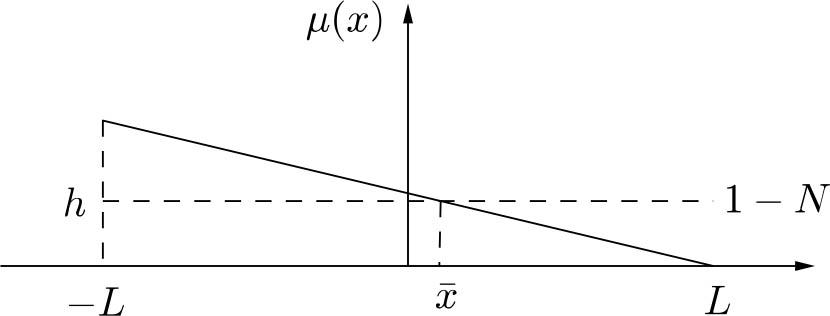Schematic representation of a mortality function for directional selection.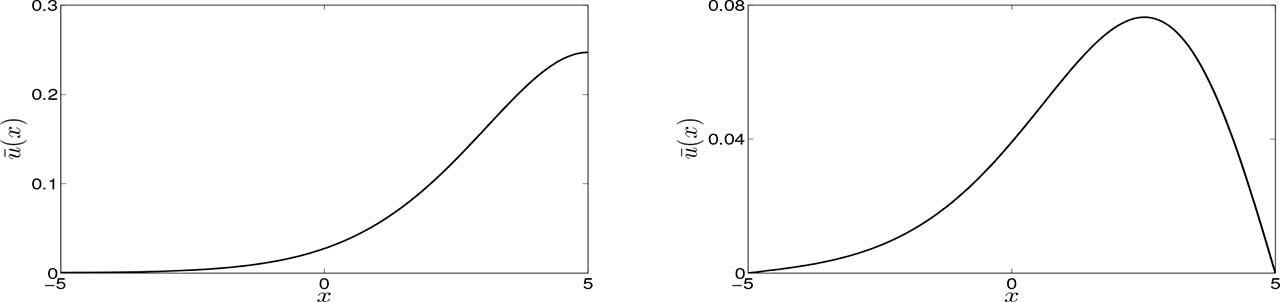Non-zero equilibrium solutions of Eq. (2) with Neumann (left) and Dirichlet (right) boundary conditions, respectively. For this simulation we consider μ(x) = 0.15(5 − x). These simulations show the typical behaviour of evolution by directional selection.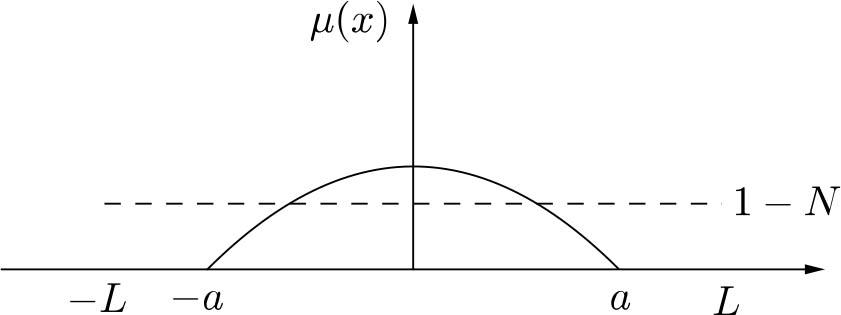Schematic representation of a mortality function for disruptive selection.
Disruptive selection

Now, let us consider the case in which the mortality rate is defined as $μ(x)=max{h(a2−x2)a2,0}, a≤L.$ \mu (x) = \max \left\{{{{h({a^2} - {x^2})} \over {{a^2}}},0} \right\},\quad a \le L.

This function describes a situation in which the extreme traits have greater adaptive value over the mean trait. That is, the mortality rate decreases with respect to distance to the mean trait. The zero phenotype has the greatest mortality rate μ(0) = h and phenotypes satisfying a ≤ |x| ≤ L have mortality rate zero.

For this function, it turns out that $12L∫Dμ(x) dx=2ah3L$ {1 \over {2L}}\int_D\mu (x)\;dx = {{2ah} \over {3L}} and thus the condition for an establishing population becomes $ah<3L2(1−θ12),$ ah < {{3L} \over 2}\left({1 - \theta_1^2} \right), which in terms of the dimensional variables turns into $ah<32(MV−π2VbM).$ ah < {3 \over 2}\left({{M \over {\sqrt V}} - {{{\pi^2}\sqrt V} \over {bM}}} \right).

In the last expression, the value of b depends on the type of boundary condition.

According to Eq. (4), we have 0 < N < 1 and, hence, 1 − N > 0. When we consider Neumann boundary conditions for Eq (2), by Proposition 2, both −L and L are critical points of the equilibrium solution. Since μ(−L) = μ(L) = 0 < 1 − N, it follows that these points are local minima of ū(x). On the other hand, since μ(x) is an even function the equilibrium solution ū must be an even function as well. Indeed, it cannot be odd, because it must be non-negative. Thus, it follows that x = 0 is a critical point of ū. According to Proposition 2 we claim that this critical point is a local minimum.

The same statement is true when we consider Dirichlet boundary conditions. That is, for a non-constant equilibrium solution, x = 0 is a local minimum for the solution ū(x). From Eq. (5) it follows that ū(−L) > 0 and ū(x) < 0 for x near zero. Thus, observing that μ(x) is increasing on (−L,0) we conclude that ū(x) has a local maximum on (−L,0). Hence, by symmetry, ū(x) has a local maximum on (0,L) as well.

In Figure 4, we see numerical solutions illustrating this behaviour.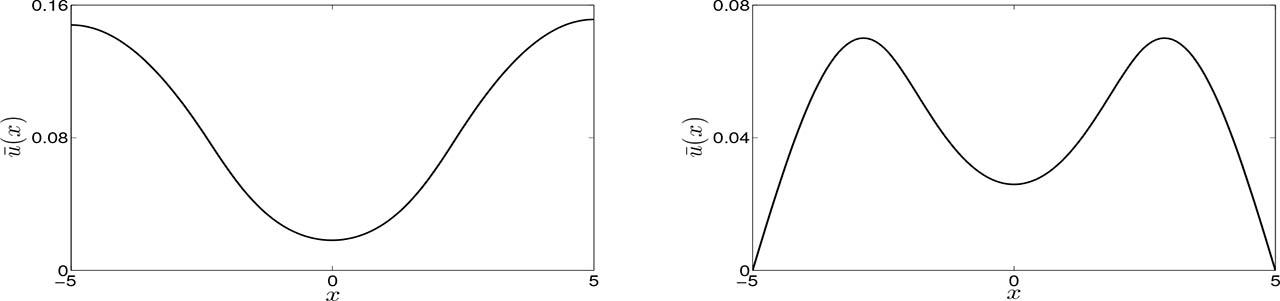Numerical simulations of nonzero equilibrium solutions for Eq. (2) with Neumann (left) and Dirichlet (right) boundary conditions, respectively. Here we consider μ(x) = max{0,0.192(6.25 − x2)}. These simulations show the typical behaviour of evolution by disruptive selection.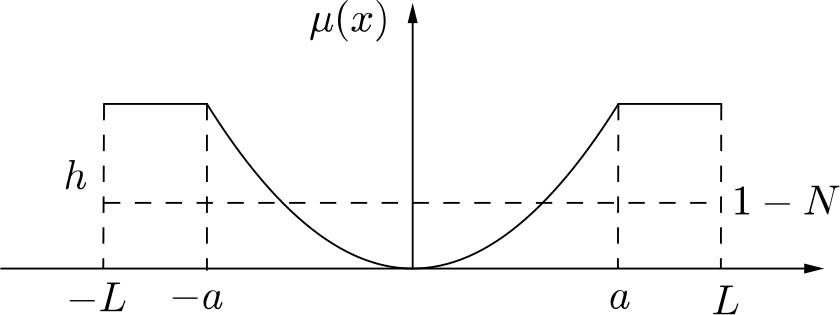Schematic representation of a mortality function for stabilising selection.
Stabilising selection

Let us consider now the situation in which the mean phenotype has the greatest adaptive value over all the others. This situation can be described by the following mortality rate function: $μ(x)=min{hx2a2,h}.$ \mu (x) = \min \left\{{{{h{x^2}} \over {{a^2}}},h} \right\}.

For this function, it is not difficult to check that $∫Dμ(x) dx=2Lh−2ah3.$ \int_D\mu (x)\;dx = 2Lh - {{2ah} \over 3}.

Now, assuming that a is a small fraction of the domain, say a = β L, then the criterion for the instability of the zero solution (16) becomes: $h(1−β3)<1−θ12.$ h\left({1 - {\beta\over 3}} \right) < 1 - \theta_1^2.

Since μ(x) is even, according to Proposition 3, the non-constant equilibrium solution has a local maximum at x = 0. Further, according to Proposition 2, for Neumann boundary conditions, both −L and L are local minima of ū(x). In Figure 6, we see numerical solutions illustrating the typical behaviour of an equilibrium solution under evolution by stabilising selection.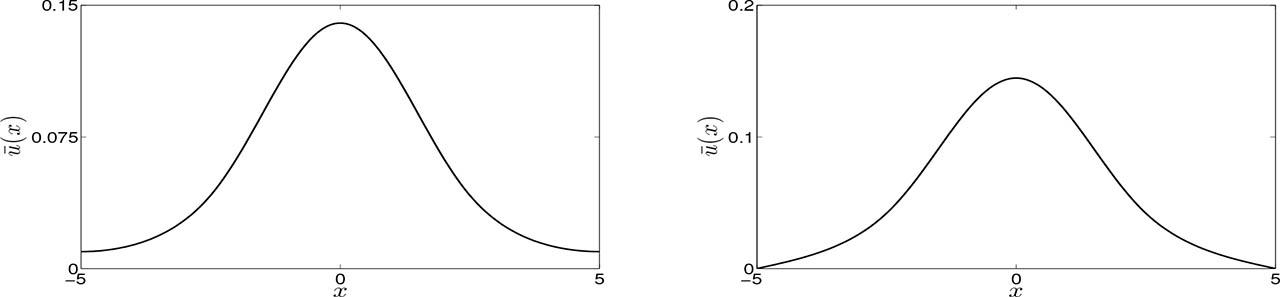Numerical simulations of nonzero equilibrium solutions for Eq. (2) with Neumann (left) and Dirichlet (right) boundary conditions, respectively. For these simulations we consider μ(x) = min{0.184x2,1.15}. These simulations show the typical evolution behaviour by stabilising selection.
Survival of the flattest

We finally address another important case of selection: the survival of the flattest. That is, we consider the case in which the fitness landscape has two peaks: one low and flat and another one higher but narrower.

In terms of mortality rates, we are interested in discussing the shape of possible equilibrium solutions when the mortality function μ(x) has two local minima: one high and flat, and the other lower but narrower (see the left frame of Figure 7).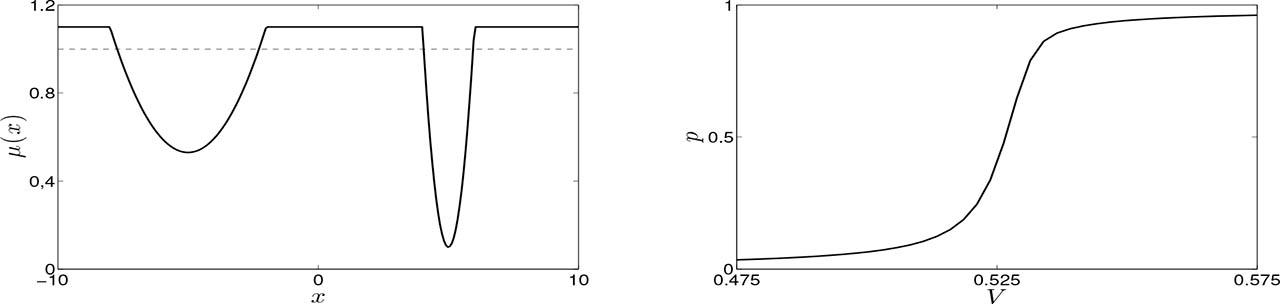Numerical simulation showing the transition from the fittest to the flattest as the mutation rate increases. Left: the function μ(x); right: the soultion for small V. Here, the horizontal line is y = 1.

As shown in previous sections, according to Proposition 2, the existence of local maximum points for an equilibrium solution relies on whether μ(x) is smaller than 1 − N or not. For this case, we have three possible scenarios: both local minima are less than 1 − N; both local minima are greater than 1 − N; just the lower local minimum is less than 1 − N. Here, we consider mainly the first scenario.

If we look at Eq. (5) we can see that the difference between μ(x) and 1−N controls the sign of the derivative of ū(x). It means that what is important here are both the size of the intervals in which μ(x) is greater or less than 1 − N as well as the difference between the mortality rate and 1 − N in each one of these intervals. Thus, at least hypothetically, we could have a non-zero equilibrium solution with either one local maximum, peaked near one of the local minima of μ(x), or two local maximum points peaked near both the local minimum points of μ(x).

However, more interesting is the role played by the mutation rate V in switching the maximum value of the equilibrium solution from one valley of μ(x) to the other. To illustrate this behaviour, consider the mortality rate function on the left in Figure 7. This function is given by $μ(x)=min{1.1,1.1+0.06(x+2)(x+8)}+min{0,(x−4)(x−6)}.$ \mu (x) = \min \left\{{1.1,1.1 + 0.06(x + 2)(x + 8)} \right\} + \min \left\{{0,(x - 4)(x - 6)} \right\}.

We also set M = 10, r = 1 and α = 1. For this mortality function, the flattest valley is on the interval [−10,0].

To measure the influence of V on the equilibrium solution ū(x), let us define p by $p=∫−M0u¯(x)dx∫−MMu¯(x)dx.$ p = {{\int_{- M}^0\bar u(x)dx} \over {\int_{- M}^M\bar u(x)dx}}.

In words, p defined as Eq. (19) measures the proportion between the number of individuals on the left over the total number of individuals. Thus, letting the mutation rate V vary we capture its influence over the equilibrium solution. In Figure 7 right, we can see that as V increases the equilibrium solutions switch from the right, i.e. a low p means more population on the rightmost half of the domain, to the left, i.e. a large p means more population on the leftmost part of the domain of the aspect space. Thus for small values of V the equilibrium solution is peaked near the minimum point of the narrower valley. Further, increasing V we clearly see the transition from the narrower valley to the flatter one, shown in Figures 8, 9.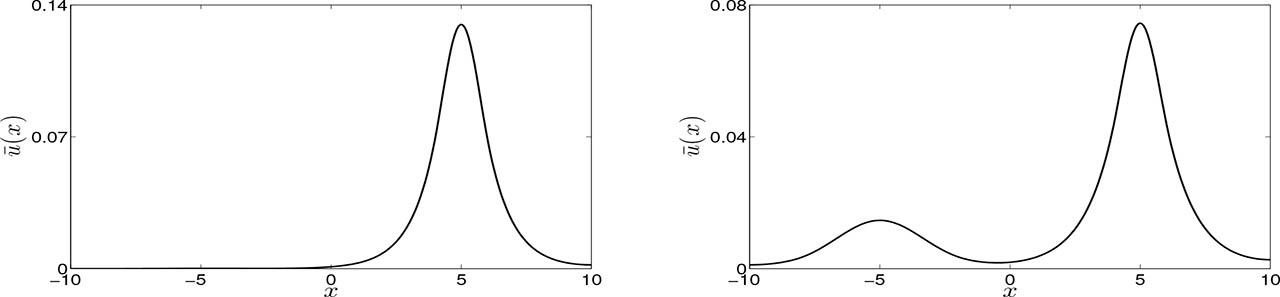Numerical simulation showing the transition from the fittest to the flattest as mutation rate increases. Left V = 0.4; right V = 0.52.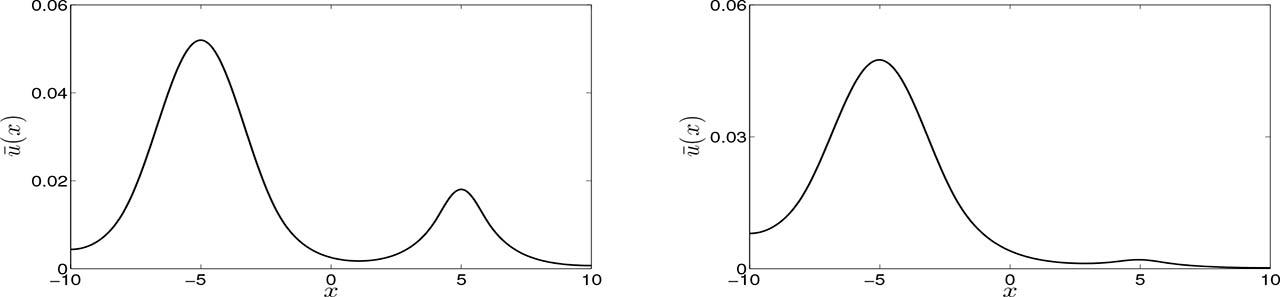Numerical simulation showing the transition from the fittest to the flattest as the mutation rate increases. Left V = 0.54; right V = 0.7.

In Figure 8 we have the graphs of two equilibrium solutions when V = 0.4 and V = 0.52. For these values of V, both equilibrium solutions have a global maximum near x = 5. However, the solution on the right has also a local maximum at x = − 5.

In Figure 9 we have the graphs of two equilibrium solutions when V = 0.54 and V = 0.7. For these values of V, both equilibrium solutions have a global maximum near x = −5. However, the solution on the left has also a local maximum at x = 5.

Conclusion

In this paper we presented some results relative to evolutionary selection through differential mortality rates. The question of population permanence has been discussed, given fixed mortality distributions and boundary conditions. Two main effects may push the population to extinction: high mutation rates leading to population dissipation or high mortality rates resulting in insufficient reproduction.

The first effect is closely related to the classical problem of critical patch size for population permanence under diffusion [4,22]. In this case, the coefficient of phenotype change, V, plays the role of a diffusion/dispersal constant. Patch size is related with how narrow the adaptation peak occupied by the biological population is. Under very narrow adaptation peaks, only species with low mutation rates can survive. This effect is present in all permanence conditions calculated in this paper. The second effect is the more obvious restriction on average mortality when compared with the reproduction rate, which can be explicitly observed in inequality (17). There is evidence that pathogens can be controlled by causing an ‘error threshold catastrophe’, by pushing mutation rates using mutagens [9, 26].

The model has shown coherence in the results of all three particular cases analysed: directional, stabilising and disruptive selection, with results mimicking qualitatively the expected and observed biological results, see Chapter 12 of . Selection of quasispecies was another characteristic displayed by the model, which is also observed in other theoretical approaches , experiments  and in an aspect space model with differential reproduction rates .

However, an important difference in the quasispecies selection based on differential mortality rates was found. The transition from the selection of one quasispecies to another one is smooth. While replicator and differential reproduction models lead to abrupt transitions from one type to another, selection through differential reproduction rates can display coexistence of types and smooth transitions from one type to another (as shown in Figure 7). Also, in this type of selection, increased mutation rates favoured the ‘selection of the flattest’, being coherent with the results obtained in other models and experiments [24, 25].

There was also an important difference observed between the dynamics of selection through mortality rates and selection based on differential reproduction rates. In the scenario with just one adaptive peak, regardless of mutation rates, selection of the fittest is observed in the model with differential mortality rates. The same cannot be said to occur in classical approaches  or even in the aspect space model with focus on reproduction rates . In such models, the mutation rate must be below a certain threshold for the fittest to be selected, a result that is coherent with biological observations.

Since mortality is just one of the many components of fitness in real systems, the results obtained by the model should not be considered as a contradiction, since a mutation rate below a certain threshold is still needed for the selection of the fittest when its other components are included. Finally, we point out that the analytical results concerning the aspect space model are original and represent the important development in understanding the dynamics of such models.

Assis, R. A., Bonelli, S., Witek, M., Barbero, F., Casacci, L., Baletto, E., Venturino, E., Jr., W. F., 2012. A model for the evolution of parasite-host interactions based on the maculinea-myrmica system: Numerical simulations and multiple host behavior. Nonlinear Analysis: Real World Applications 13, 1507–1524. AssisR. A. BonelliS. WitekM. BarberoF. CasacciL. BalettoE. VenturinoE.Jr. W. F. 2012 A model for the evolution of parasite-host interactions based on the maculinea-myrmica system: Numerical simulations and multiple host behavior Nonlinear Analysis: Real World Applications 13 1507 1524 10.1016/j.nonrwa.2011.10.008 Search in Google Scholar

Assis, R. A., Ferreira Jr., W. C., 2016. A reaction-diffusion model for phenotypic evolution. Computational and Applied Mathematics In press. AssisR. A. FerreiraW. C.Jr. 2016 A reaction-diffusion model for phenotypic evolution Computational and Applied Mathematics In press Search in Google Scholar

Barton, N. H., Briggs, D. E. G., Eisen, J. A., Goldstein, D. B., Patel, N. H., 2007. Evolution. Cold Spring Harbor Laboratory Press, New York. BartonN. H. BriggsD. E. G. EisenJ. A. GoldsteinD. B. PatelN. H. 2007 Evolution Cold Spring Harbor Laboratory Press New York Search in Google Scholar

Ben-Yu, G., Mitchell, A. R., Sleeman, B. D., 1986. Spatial effects in a two-dimensional model of the budworm-balsam fir ecosystem. Comp. and Maths. with Appls. 12B, 1117–1132. Ben-YuG. MitchellA. R. SleemanB. D. 1986 Spatial effects in a two-dimensional model of the budworm-balsam fir ecosystem Comp. and Maths. with Appls. 12B 1117 1132 10.1016/0898-1221(86)90236-1 Search in Google Scholar

Blagosklonny, M. V., 2010. Revisiting the antagonistic pleiotropy theory of aging tor-driven program and quasi-program. Cell Cycle 9, 3151–3156. BlagosklonnyM. V. 2010 Revisiting the antagonistic pleiotropy theory of aging tor-driven program and quasi-program Cell Cycle 9 3151 3156 10.4161/cc.9.16.1312020724817 Search in Google Scholar

Bourguet, D., Prout, M., Raymond, M., 1996. Dominance of insecticide resistance presents a plastic response. Genetics 143, 407–416. BourguetD. ProutM. RaymondM. 1996 Dominance of insecticide resistance presents a plastic response Genetics 143 407 416 10.1093/genetics/143.1.40712072738722792 Search in Google Scholar

Brodie III, E. D., Brodie Jr., E. D., 1999. Predator-prey arms race: asymmetrical selection on predators and prey may be reduced when prey are dangerous. Biosciences 49, 557–568. BrodieE. D.III BrodieE. D.Jr. 1999 Predator-prey arms race: asymmetrical selection on predators and prey may be reduced when prey are dangerous Biosciences 49 557 568 10.2307/1313476 Search in Google Scholar

Codoner, F. M., Darós, J. A., Solé, R. V., Elena, S. F., 2006. The fittest versus the flattest: Experimental confirmation of the quasispecies effect with subviral pathogens. PLoS Pathogens 2, 1187–1192. CodonerF. M. DarósJ. A. SoléR. V. ElenaS. F. 2006 The fittest versus the flattest: Experimental confirmation of the quasispecies effect with subviral pathogens PLoS Pathogens 2 1187 1192 10.1371/journal.ppat.0020136175720317196038 Search in Google Scholar

Crotty, S., Cameron, C. E., Andino, R., 2001. Rna virus error catastrophe: Direct molecular test by using ribavirin. PNAS 98, 6895–6900. CrottyS. CameronC. E. AndinoR. 2001 Rna virus error catastrophe: Direct molecular test by using ribavirin PNAS 98 6895 6900 10.1073/pnas.1110855983444911371613 Search in Google Scholar

Dawkins, R., 1976. The Selfish Gene. Oxford University Press, Oxford. DawkinsR. 1976 The Selfish Gene Oxford University Press Oxford Search in Google Scholar

Dawkins, R., 1982. The Extended Phenotype. Oxford University Press, Oxford. DawkinsR. 1982 The Extended Phenotype Oxford University Press Oxford Search in Google Scholar

Desmond, A., Moore, J., 1991. Darwin: The Life of a Tormented Evolucionist. Norton, New York. DesmondA. MooreJ. 1991 Darwin: The Life of a Tormented Evolucionist Norton New York Search in Google Scholar

Dilda, C. L., Mackay, T. F. C., 2002. The genetic architecture of the drosophila sensory bristle number. Genetics 162, 1655–1674. DildaC. L. MackayT. F. C. 2002 The genetic architecture of the drosophila sensory bristle number Genetics 162 1655 1674 10.1093/genetics/162.4.1655146236312524340 Search in Google Scholar

Fisher, R. A., 1930. The Genetical Theory of Natural Selection. Dover Publications, New York. FisherR. A. 1930 The Genetical Theory of Natural Selection Dover Publications New York 10.5962/bhl.title.27468 Search in Google Scholar

Futuyma, D. J., 2009. Evolution. Sinauer Associates Publishers Inc., Sunderland. FutuymaD. J. 2009 Evolution Sinauer Associates Publishers Inc. Sunderland Search in Google Scholar

Gillespie, J. H., 2004. Population Genetics: A Concise Guide. John Hopkins University Press, London. GillespieJ. H. 2004 Population Genetics: A Concise Guide John Hopkins University Press London Search in Google Scholar

Gould, S. J., 2002. The Structure of Evolutionary Theory. The Belknap Press of Harvard University Press, London, England. GouldS. J. 2002 The Structure of Evolutionary Theory The Belknap Press of Harvard University Press London, England Search in Google Scholar

Hamilton, W. D., 1963. The evolution of altruistic behavior. American Naturalist 97, 354–356. HamiltonW. D. 1963 The evolution of altruistic behavior American Naturalist 97 354 356 10.1086/497114 Search in Google Scholar

Hartl, D. L., Clark, A. G., 2007. Principles of Population Genetics. Sinauer Associates Publishers Inc., Sunderland. HartlD. L. ClarkA. G. 2007 Principles of Population Genetics Sinauer Associates Publishers Inc. Sunderland Search in Google Scholar

Levins, R., Lewotin, R., 1980. The Dialectical Biologist. Harvard University Press, Cambridge. LevinsR. LewotinR. 1980 The Dialectical Biologist Harvard University Press Cambridge Search in Google Scholar

Lewotin, R., 1970. The units of selection. Journal of Mathematical Biology 1, 1–18. LewotinR. 1970 The units of selection Journal of Mathematical Biology 1 1 18 10.1146/annurev.es.01.110170.000245 Search in Google Scholar

Ludwig, D., Aronson, G. D., Weinberger, H. F., 1979. Spatial patterning of the spruce budworm. Journal of Mathematical Biology 8, 217–258. LudwigD. AronsonG. D. WeinbergerH. F. 1979 Spatial patterning of the spruce budworm Journal of Mathematical Biology 8 217 258 10.1007/BF00276310 Search in Google Scholar

Murray, J. D., 1989. Mathematical Biology. Springer, New York. MurrayJ. D. 1989 Mathematical Biology Springer New York 10.1007/978-3-662-08539-4 Search in Google Scholar

Nowak, M. A., 1992. What is a quasispecies? Trends in Ecology & Evolution 7, 118–121. NowakM. A. 1992 What is a quasispecies? Trends in Ecology & Evolution 7 118 121 10.1016/0169-5347(92)90145-2 Search in Google Scholar

Nowak, M. A., 2006. Evolutionary dynamics: exploring the equations of life. Harvard University Press, Cambridge. NowakM. A. 2006 Evolutionary dynamics: exploring the equations of life Harvard University Press Cambridge 10.2307/j.ctvjghw98 Search in Google Scholar

Pfeiffer, J. K., Kirkegaard, K., 2003. A single mutation in poliovirus rna-dependent rna polymerase confers resistance to mutagenic nucleotide analogs via increased fidelity. PNAS 100, 7289–7294. PfeifferJ. K. KirkegaardK. 2003 A single mutation in poliovirus rna-dependent rna polymerase confers resistance to mutagenic nucleotide analogs via increased fidelity PNAS 100 7289 7294 10.1073/pnas.1232294100 Search in Google Scholar

Phillips, B. L., Shine, R., 2004. Adapting to an invasive species: toxic cane toads induce morphological change in australian snakes. PNAS 101, 17150–17155. PhillipsB. L. ShineR. 2004 Adapting to an invasive species: toxic cane toads induce morphological change in australian snakes PNAS 101 17150 17155 10.1073/pnas.0406440101 Search in Google Scholar

Smith, J. M., 1982. Evolution and the Theory of Games. Cambridge University Press, Cambridge. SmithJ. M. 1982 Evolution and the Theory of Games Cambridge University Press Cambridge 10.1017/CBO9780511806292 Search in Google Scholar

Taylor, C., Higgs, P., 2000. A population genetics model for multiple quantitative traits exhibiting pleiotropy and epistasis. Journal of Theoretical Biology 203, 419–437. TaylorC. HiggsP. 2000 A population genetics model for multiple quantitative traits exhibiting pleiotropy and epistasis Journal of Theoretical Biology 203 419 437 10.1006/jtbi.2000.1094 Search in Google Scholar

Taylor, P. D., Jonker, L. B., 1978. Evolutionary stable strategies and game dynamics. Mathematical Biosciences 40, 145 – 156. TaylorP. D. JonkerL. B. 1978 Evolutionary stable strategies and game dynamics Mathematical Biosciences 40 145 156 10.1016/0025-5564(78)90077-9 Search in Google Scholar

• #### Higher Education Agglomeration Promoting Innovation and Entrepreneurship Based on Spatial Dubin Model

Articles recommandés par Trend MD